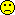## Who can help? [🇷 for BE/BA]

Hi all,

some help needed.Specifically, I am asking for help to make code work and not to make it smarter (achieving the same with less key strokes, using built-in alternatives etc) although there is generally a preferred focus on the latter hereHopefully we will discuss later the need for additional or other variance components once we have made the optimiser work somehow (or while we are making it work, if such vc's are totally necessary). There are probably a ton of errors to iron out. There is at least one big one whose effects I a sufferingThe idea is to construct the model from the bottoms up.
1. First I do a full-rank design matrix X for the fixed effects, without subjects
2. Next I do the covariance matrix with just three variance components as discussed above.
3. I generate some cheap starter guesses for the variance components
4. I define an objective function for "a constant + log likelihood"
5. I optimise the variance components, in REML the effect estimates will come along with them.
I am so far facing a singularity with the apparent log likelihood, so obviously something is terribly wrong. I include a ref to the function where I got inspiration for the log likelihood objective function.
Another site for the objective function is: https://www.stt.msu.edu/users/pszhong/Lecture_16_Spring_2017.pdf (page 13+14), but this also did not quite get me to convergence.

So, this is what I have so far. I don't understand matrix algebra totally well, and I am at a loss of you say Hermitian, expectation-minimisation, Jacobian, etc.

What bothers me is that some years ago I wrote a working optimiser in C on basis of slides some some Danish guy called Waagepetersen, who walked the reader slowly through the process of optimising REML. I can't find my code now, it was on another computer, and I can't find his slides any more (there are some slides with his name out there, which I can find with google etc but they do not have that old recipe).

rm(list=ls(all=TRUE)) ###### par vector is c(varT, varBR, varWR) ###### ######   Section 1: Household things ###### CreateX=function(D) {  ##first the two treatments  TrtT=as.numeric(as.character(D$Trt)=="T") TrtR=as.numeric(as.character(D$Trt)=="R")  X=cbind(TrtT, TrtR)  R= qr(X)$rank ## subjects: in a mixed model with subj as random ## we do not do subjects also as fixed, therefore they are #'ed away here ## for (s in unique(D$Subj))  ## {  ## v=as.numeric(D$Subj==s) ## #print(v) ## XX=data.frame(X, v) ## names(XX)[ncol(XX)] = paste("S", s, sep="") ## rnk=qr(XX)$rank  ## if (rnk>R)   ##  {   ##     X=XX   ##     R=rnk   ##  }   ## }  ##now the Pers  for (p in unique(D$Per)) { v=as.numeric(D$Per==p)   #print(v)   XX=data.frame(X, v)   names(XX)[ncol(XX)] = paste("P", p, sep="")   rnk=qr(XX)$rank if (rnk>R) { X=XX R=rnk } } for (q in unique(D$Seq))  {   v=as.numeric(D$Seq==q) #print(v) XX=data.frame(X, v) names(XX)[ncol(XX)] = paste("Q", q, sep="") rnk=qr(XX)$rank   if (rnk>R)     {        X=XX        R=rnk     }  }  return(as.matrix(X)) } Create.CovM=function(Params) ##block diagonal covariance matrix {   varT=Params   varBR=Params   varWR=Params   #varRT=Params   #cat("Vars:", varT, varBR, varWR,"\n")   Nobs=length(D$Y) V=matrix(0,ncol=Nobs, nrow=Nobs) for (iRow in 1:Nobs) for (iCol in 1:Nobs) { ## the diagonal if (iCol==iRow) { if (D$Trt[iRow]=="T") V[iRow,iCol]=V[iRow,iCol]+varT       if (D$Trt[iRow]=="R") V[iRow,iCol]=V[iRow,iCol]+varWR } ## off diagonal if (iCol!=iRow) if (D$Subj[iRow]==D$Subj[iCol]) { if (D$Trt[iCol]==D$Trt[iRow]) V[iRow,iCol]= V[iRow,iCol]+varBR #if (D$Trt[iCol]!=D$Trt[iRow]) V[iRow,iCol]= V[iRow,iCol]+varRT } } return(as.matrix(V)) } ###### ###### Section 2: Matrix things ###### Obj.F12=function(Pars) ##this is line 3 of page 10 of: ##http://people.csail.mit.edu/xiuming/docs/tutorials/reml.pdf { CovM=Create.CovM(Pars) A= -0.5*log(det(CovM)) B= -0.5*log(det(t(X) %*% solve(CovM) %*% X)) est.b = solve(t(X) %*% solve(CovM) %*% X) %*% t(X) %*% solve(CovM) %*% y tmp= y - X %*% est.b C=-0.5 *(t(tmp) %*% solve(CovM) %*% tmp) return(A+B+C) } Some.Initial.Guesses=function(foo) { #guess variance within R D1=subset(D, D$Trt=="R")   m=lm(log(Y)~factor(Subj)+factor(Seq)+factor(Per) , data=D1)   #print(anova(m))   varWR= anova(m)["Residuals", "Mean Sq"]   m=lm(log(Y)~factor(Subj)+factor(Seq)+factor(Per)+factor(Trt) , data=D)   varBR= anova(m)["factor(Subj)", "Mean Sq"]   ##total var T, cheaply   ##actually, this may be much better: varT=varBR+varWR   D2=subset(D, D$Trt=="T") varT=var(log(D2$Y))   varT=varBR+varWR   L=list(varT=varT, varBR=varBR, varWR=varWR)   return(L) } #### #### section 3: execution #### D=read.csv("EMAII.a.csv" , header=T, sep="\t") ##public = easier X=CreateX(D) ##public = easier y=log(D$Y) ##public = easier Check.Surf=function(i, x1,x2, N) ## Checks the log likelihood surface of the i'th variance component ## (given the other variance components) for the interval var = x1 to x2 ## with a granularity of N points. { L=Some.Initial.Guesses(0) parIni=c(L$varT, L$varBR, L$varWR )   x=x1;   vX=NULL   vY=NULL   dx=(x2-x1)/N   for (j in 1:N)   {     vX=c(vX, x)     parIni[i]=x     vY=c(vY, Obj.F12(parIni))     x=x+dx   }   plot(vX, vY) } MyREML=function(foo.bar) ## ideally this would work, but it doesn't right now## also, I have a feeling the surface may be quite rugged, ## so I may switch to a homecooked optimiser ## once I have ironed out the singularity issue, see below. {   L=Some.Initial.Guesses(0)   print(L)   parIni=c(L$varT, L$varBR, L\$varWR)   F=optim(par=parIni, fn=Obj.F12, #method="L-BFGS-B",lower=c(0,0,0),       control=list(reltol=1e-10, trace=T))   print(F) } # if ti worked it'd be called through # MyREML(0) # however: Check.Surf(1, 0.01, 0.2, 50)   ## ouch, that's a singularity.   ## so something is wrong in the covariance matrix   ## or in the "const + likelihood function"                        ## or elsewhere. Any ideas? # # #

Pass or fail!
ElMaestroIng. Helmut Schütz